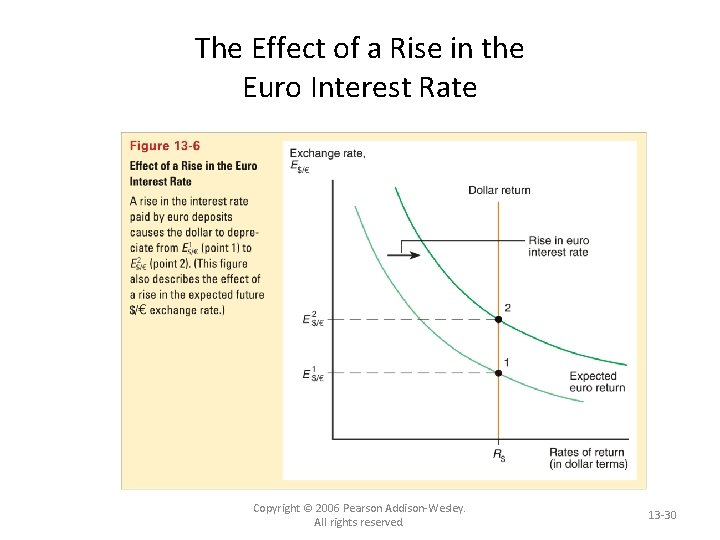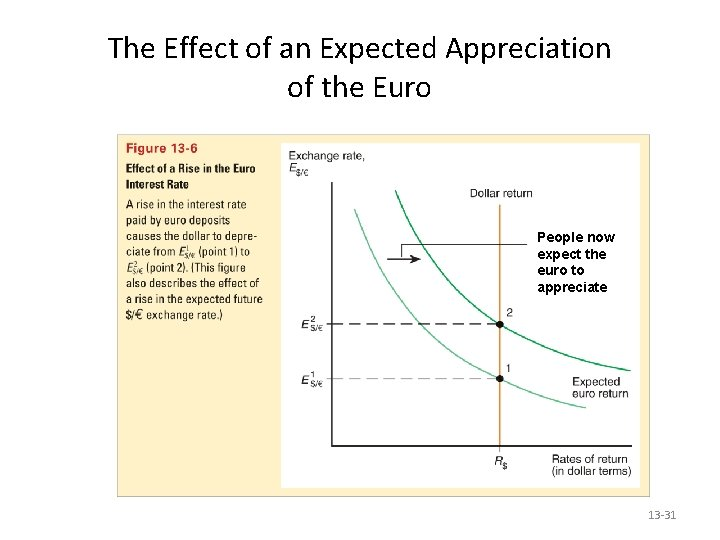# Chapter 13 Exchange Rates and the Foreign Exchange

• Slides: 31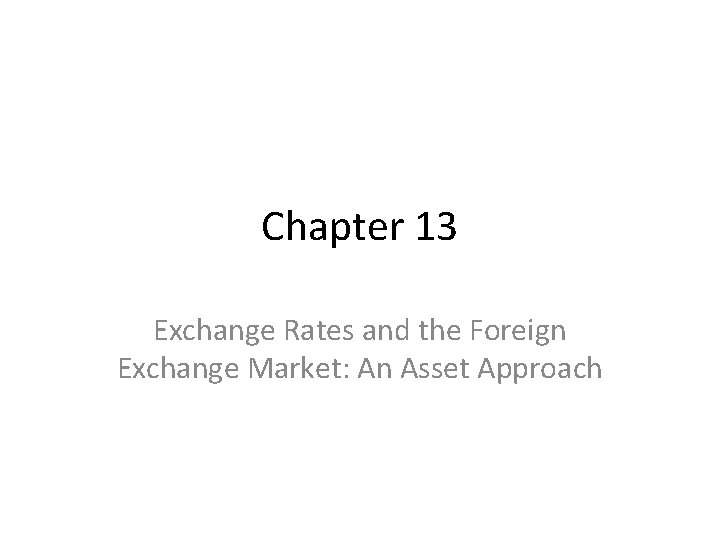Chapter 13 Exchange Rates and the Foreign Exchange Market: An Asset Approach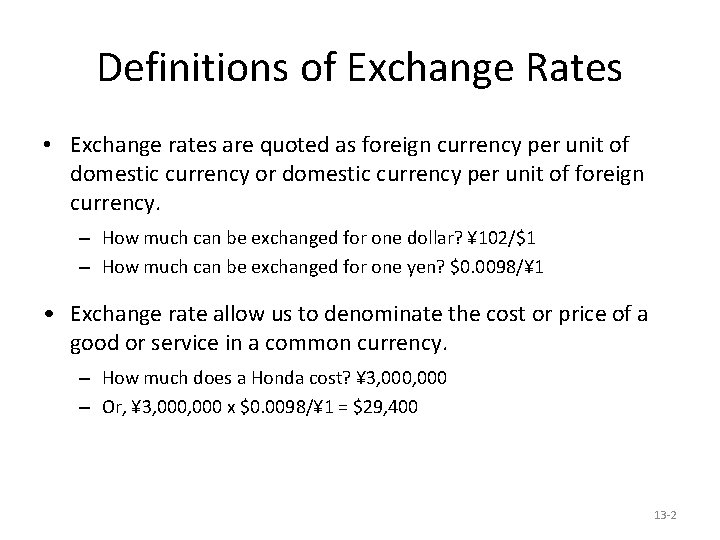Definitions of Exchange Rates • Exchange rates are quoted as foreign currency per unit of domestic currency or domestic currency per unit of foreign currency. – How much can be exchanged for one dollar? ¥ 102/\$1 – How much can be exchanged for one yen? \$0. 0098/¥ 1 • Exchange rate allow us to denominate the cost or price of a good or service in a common currency. – How much does a Honda cost? ¥ 3, 000 – Or, ¥ 3, 000 x \$0. 0098/¥ 1 = \$29, 400 13 -2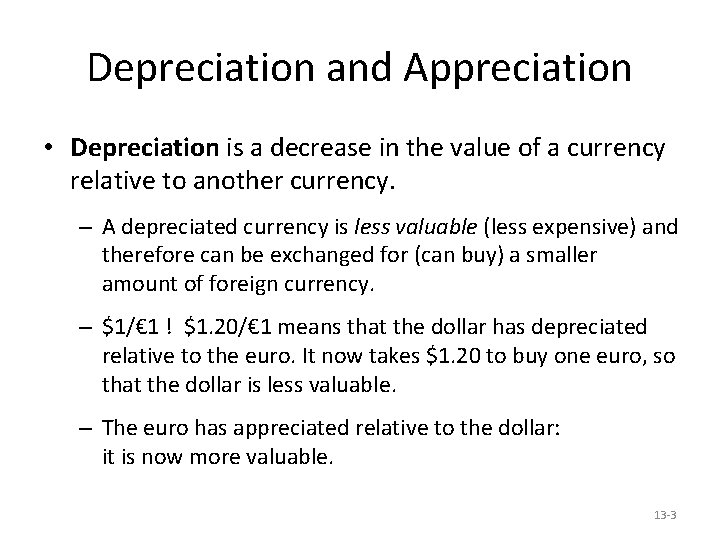Depreciation and Appreciation • Depreciation is a decrease in the value of a currency relative to another currency. – A depreciated currency is less valuable (less expensive) and therefore can be exchanged for (can buy) a smaller amount of foreign currency. – \$1/€ 1 ! \$1. 20/€ 1 means that the dollar has depreciated relative to the euro. It now takes \$1. 20 to buy one euro, so that the dollar is less valuable. – The euro has appreciated relative to the dollar: it is now more valuable. 13 -3Depreciation and Appreciation (cont. ) • Appreciation is an increase in the value of a currency relative to another currency. – An appreciated currency is more valuable (more expensive) and therefore can be exchanged for (can buy) a larger amount of foreign currency. – \$1/€ 1 ! \$0. 90/€ 1 means that the dollar has appreciated relative to the euro. It now takes only \$0. 90 to buy one euro, so that the dollar is more valuable. – The euro has depreciated relative to the dollar: it is now less valuable. 13 -4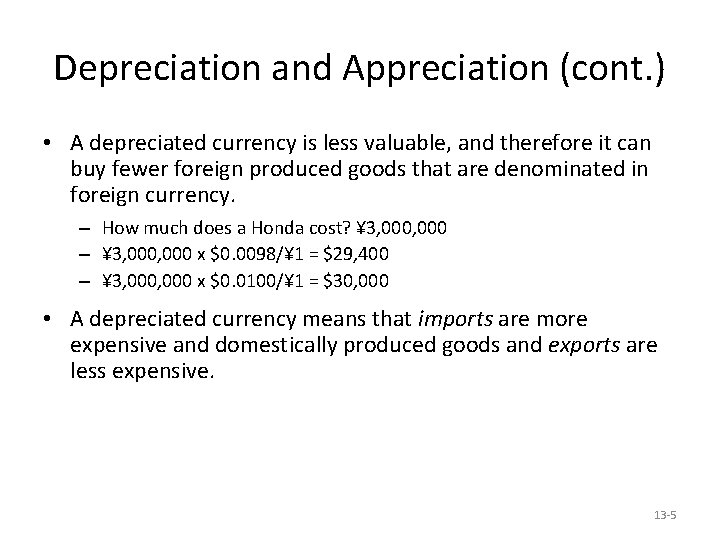Depreciation and Appreciation (cont. ) • A depreciated currency is less valuable, and therefore it can buy fewer foreign produced goods that are denominated in foreign currency. – How much does a Honda cost? ¥ 3, 000 – ¥ 3, 000 x \$0. 0098/¥ 1 = \$29, 400 – ¥ 3, 000 x \$0. 0100/¥ 1 = \$30, 000 • A depreciated currency means that imports are more expensive and domestically produced goods and exports are less expensive. 13 -5Depreciation and Appreciation (cont. ) • An appreciated currency is more valuable, and therefore it can buy more foreign produced goods that are denominated in foreign currency. – How much does a Honda cost? ¥ 3, 000 – ¥ 3, 000 x \$0. 0098/¥ 1 = \$29, 400 – ¥ 3, 000 x \$0. 0090/¥ 1 = \$27, 000 • An appreciated currency means that imports are less expensive and domestically produced goods and exports are more expensive. 13 -6The Foreign Exchange Market The participants: 1. Commercial banks and other depository institutions: transactions involve buying/selling of bank deposits in different currencies for investment. 2. Non bank financial institutions (pension funds, insurance funds) may buy/sell foreign assets. 3. Private firms: conduct foreign currency transactions to buy/sell goods, assets or services. 4. Central banks: conduct official international reserves transactions. 13 -7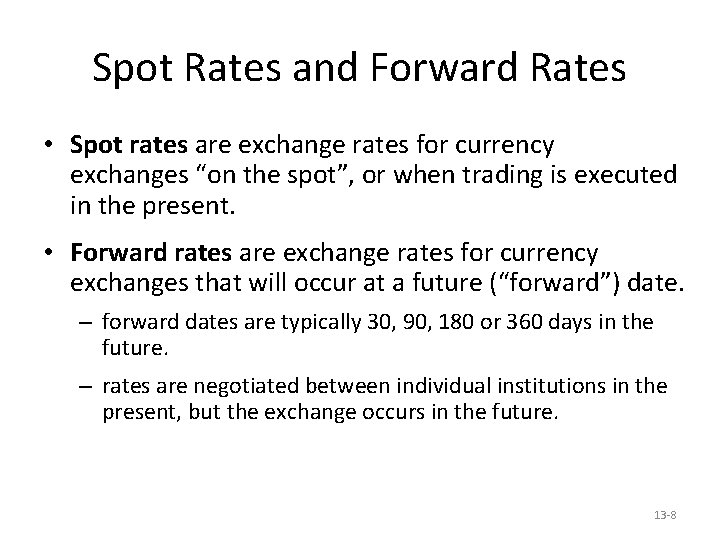Spot Rates and Forward Rates • Spot rates are exchange rates for currency exchanges “on the spot”, or when trading is executed in the present. • Forward rates are exchange rates for currency exchanges that will occur at a future (“forward”) date. – forward dates are typically 30, 90, 180 or 360 days in the future. – rates are negotiated between individual institutions in the present, but the exchange occurs in the future. 13 -8Spot and Forward Rates 13 -9The Demand for Currency Deposits • What influences the demand for (willingness to buy) deposits denominated in domestic or foreign currency? • Factors that influence the return on assets determine the demand for those assets. 13 -10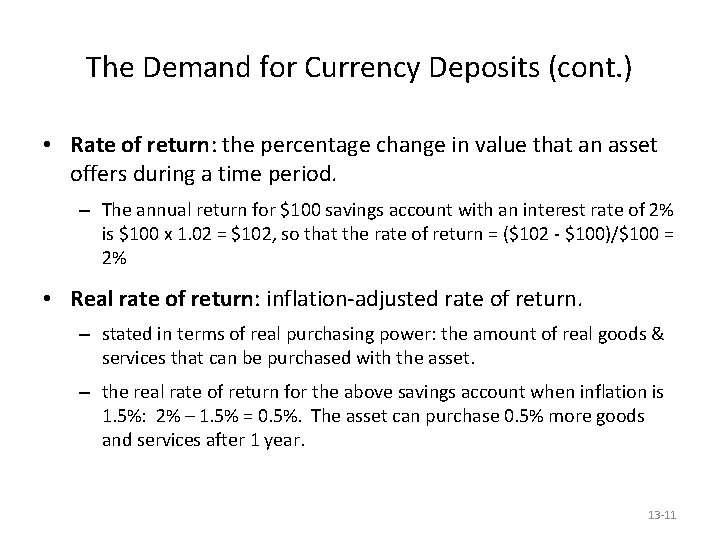The Demand for Currency Deposits (cont. ) • Rate of return: the percentage change in value that an asset offers during a time period. – The annual return for \$100 savings account with an interest rate of 2% is \$100 x 1. 02 = \$102, so that the rate of return = (\$102 - \$100)/\$100 = 2% • Real rate of return: inflation-adjusted rate of return. – stated in terms of real purchasing power: the amount of real goods & services that can be purchased with the asset. – the real rate of return for the above savings account when inflation is 1. 5%: 2% – 1. 5% = 0. 5%. The asset can purchase 0. 5% more goods and services after 1 year. 13 -11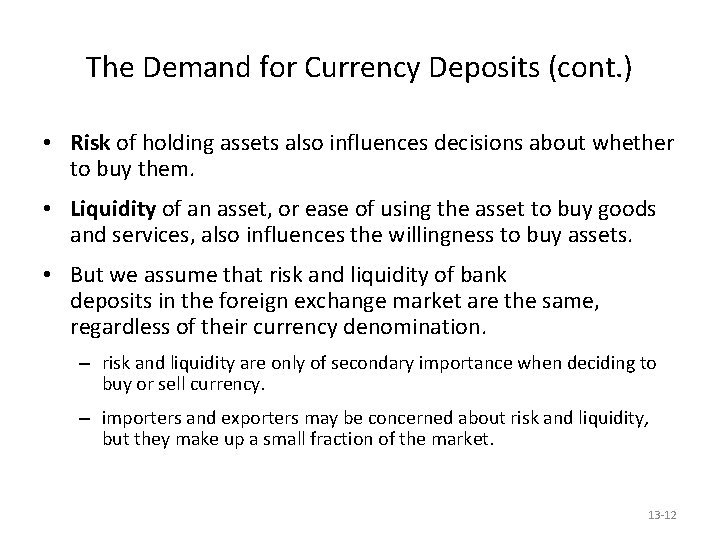The Demand for Currency Deposits (cont. ) • Risk of holding assets also influences decisions about whether to buy them. • Liquidity of an asset, or ease of using the asset to buy goods and services, also influences the willingness to buy assets. • But we assume that risk and liquidity of bank deposits in the foreign exchange market are the same, regardless of their currency denomination. – risk and liquidity are only of secondary importance when deciding to buy or sell currency. – importers and exporters may be concerned about risk and liquidity, but they make up a small fraction of the market. 13 -12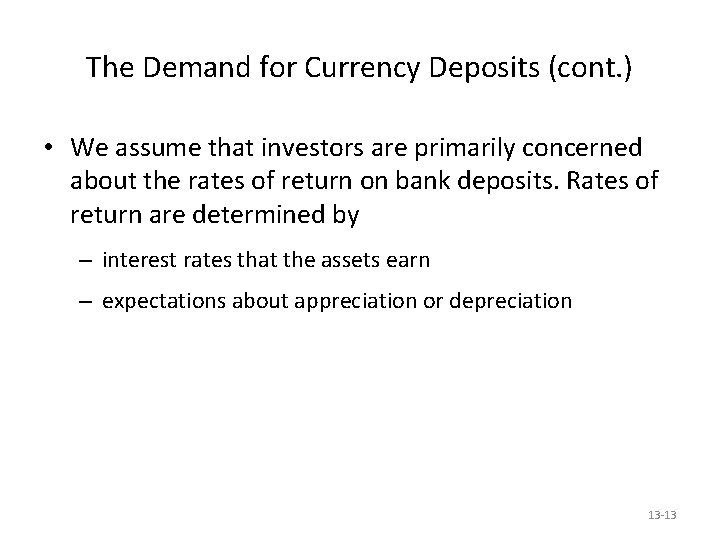The Demand for Currency Deposits (cont. ) • We assume that investors are primarily concerned about the rates of return on bank deposits. Rates of return are determined by – interest rates that the assets earn – expectations about appreciation or depreciation 13 -13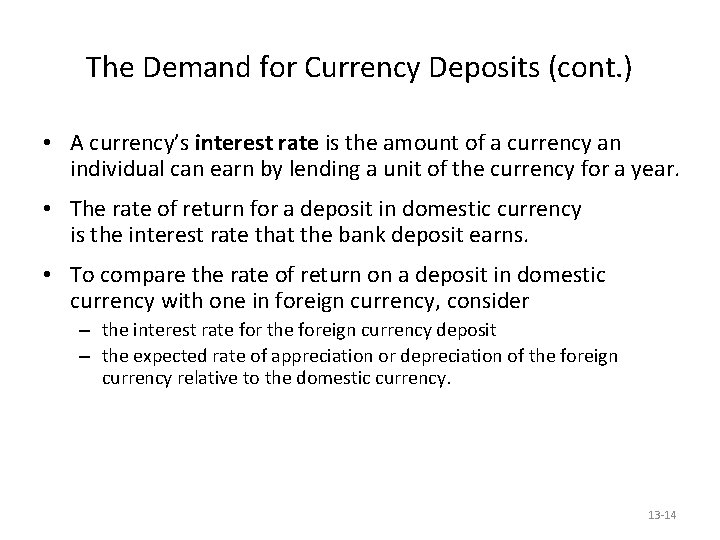The Demand for Currency Deposits (cont. ) • A currency’s interest rate is the amount of a currency an individual can earn by lending a unit of the currency for a year. • The rate of return for a deposit in domestic currency is the interest rate that the bank deposit earns. • To compare the rate of return on a deposit in domestic currency with one in foreign currency, consider – the interest rate for the foreign currency deposit – the expected rate of appreciation or depreciation of the foreign currency relative to the domestic currency. 13 -14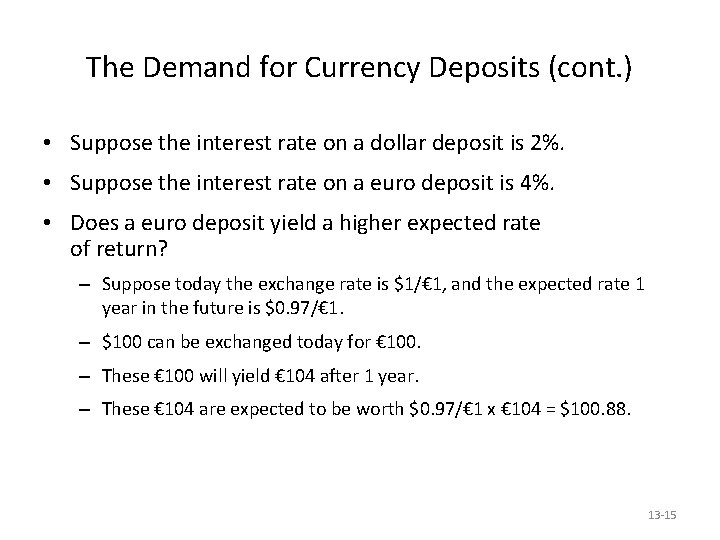The Demand for Currency Deposits (cont. ) • Suppose the interest rate on a dollar deposit is 2%. • Suppose the interest rate on a euro deposit is 4%. • Does a euro deposit yield a higher expected rate of return? – Suppose today the exchange rate is \$1/€ 1, and the expected rate 1 year in the future is \$0. 97/€ 1. – \$100 can be exchanged today for € 100. – These € 100 will yield € 104 after 1 year. – These € 104 are expected to be worth \$0. 97/€ 1 x € 104 = \$100. 88. 13 -15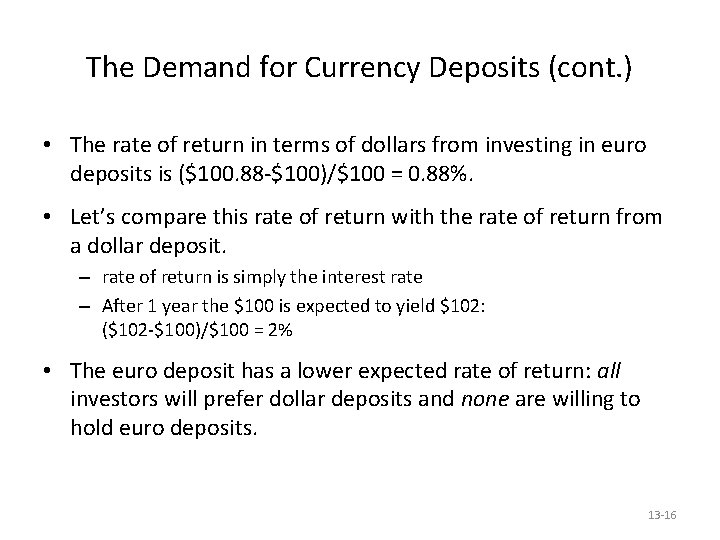The Demand for Currency Deposits (cont. ) • The rate of return in terms of dollars from investing in euro deposits is (\$100. 88 -\$100)/\$100 = 0. 88%. • Let’s compare this rate of return with the rate of return from a dollar deposit. – rate of return is simply the interest rate – After 1 year the \$100 is expected to yield \$102: (\$102 -\$100)/\$100 = 2% • The euro deposit has a lower expected rate of return: all investors will prefer dollar deposits and none are willing to hold euro deposits. 13 -16The Demand for Currency Deposits (cont. ) • Note that the expected rate of appreciation of the euro is (\$0. 97 - \$1)/\$1 = -0. 03 = -3%. • We simplify the analysis by saying that the dollar rate of return on euro deposits approximately equals – the interest rate on euro deposits – plus the expected rate of appreciation on euro deposits – 4% + -3% = 1% ≈ 0. 88% • R€ + (Ee\$/€ - E\$/€)/E\$/€ 13 -17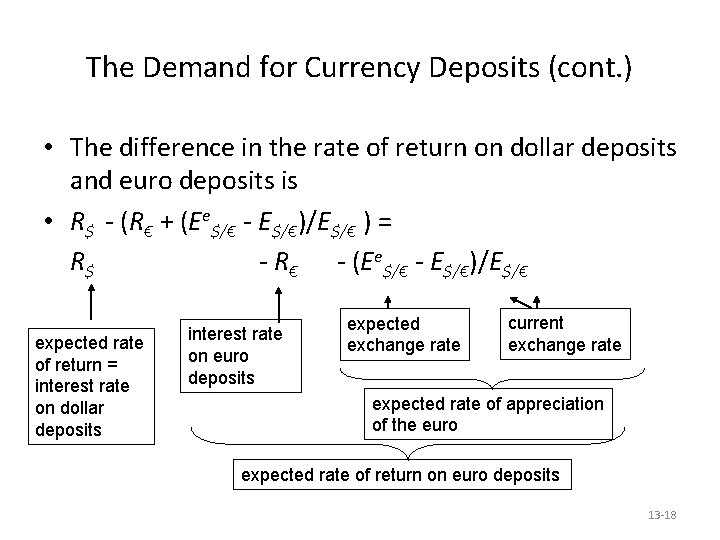The Demand for Currency Deposits (cont. ) • The difference in the rate of return on dollar deposits and euro deposits is • R\$ - (R€ + (Ee\$/€ - E\$/€)/E\$/€ ) = R\$ - R€ - (Ee\$/€ - E\$/€)/E\$/€ expected rate of return = interest rate on dollar deposits interest rate on euro deposits expected exchange rate current exchange rate expected rate of appreciation of the euro expected rate of return on euro deposits 13 -18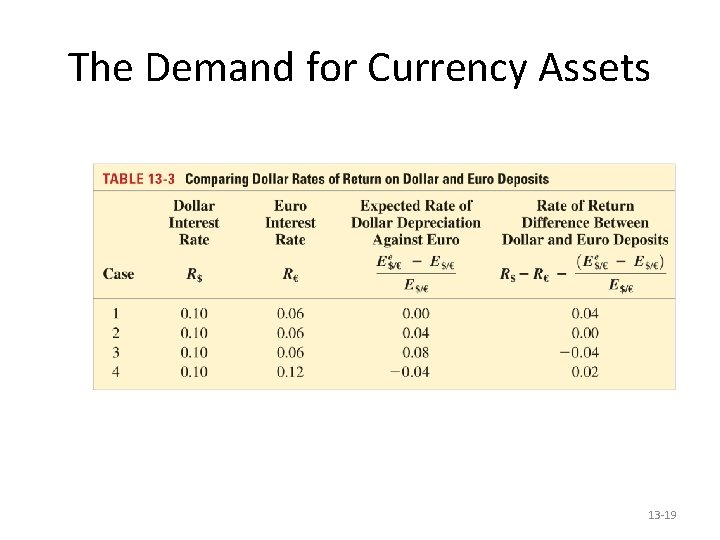The Demand for Currency Assets 13 -19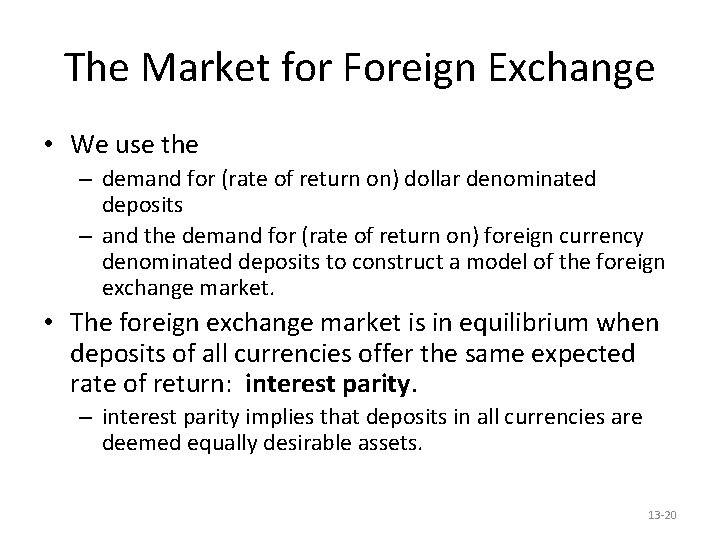The Market for Foreign Exchange • We use the – demand for (rate of return on) dollar denominated deposits – and the demand for (rate of return on) foreign currency denominated deposits to construct a model of the foreign exchange market. • The foreign exchange market is in equilibrium when deposits of all currencies offer the same expected rate of return: interest parity. – interest parity implies that deposits in all currencies are deemed equally desirable assets. 13 -20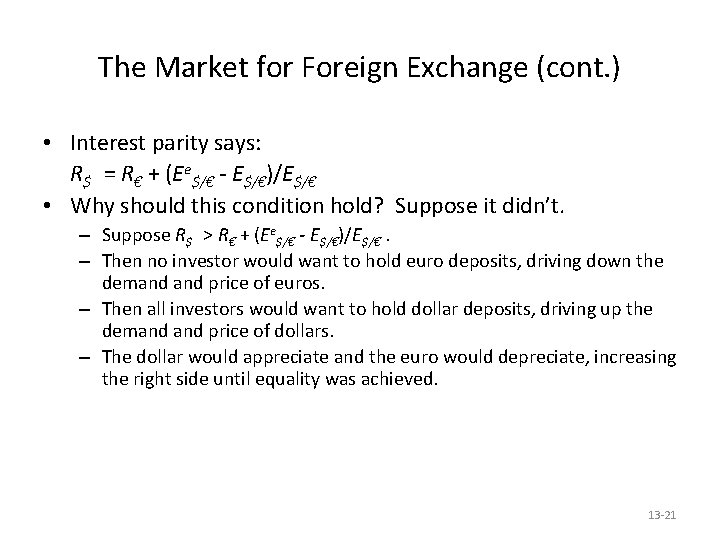The Market for Foreign Exchange (cont. ) • Interest parity says: R\$ = R€ + (Ee\$/€ - E\$/€)/E\$/€ • Why should this condition hold? Suppose it didn’t. – Suppose R\$ > R€ + (Ee\$/€ - E\$/€)/E\$/€. – Then no investor would want to hold euro deposits, driving down the demand price of euros. – Then all investors would want to hold dollar deposits, driving up the demand price of dollars. – The dollar would appreciate and the euro would depreciate, increasing the right side until equality was achieved. 13 -21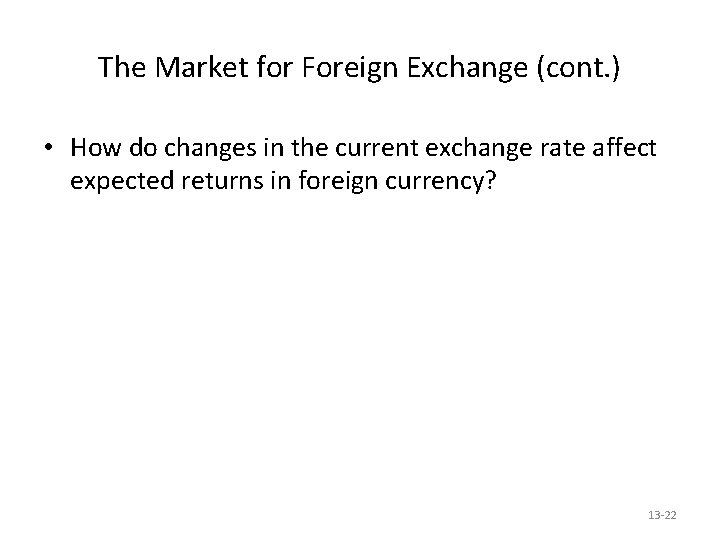The Market for Foreign Exchange (cont. ) • How do changes in the current exchange rate affect expected returns in foreign currency? 13 -22The Market for Foreign Exchange (cont. ) • Depreciation of the domestic currency today lowers the expected return on deposits in foreign currency. – A current depreciation of domestic currency will raise the initial cost of investing in foreign currency, thereby lowering the expected return in foreign currency. • Appreciation of the domestic currency today raises the expected return of deposits in foreign currency. – A current appreciation of the domestic currency will lower the initial cost of investing in foreign currency, thereby raising the expected return in foreign currency. 13 -23Expected Returns on Euro Deposits when Ee\$/€ = \$1. 05 Per Euro Expected rate of Current Interest rate on dollar exchange rate euro deposits depreciation E\$/€ R€ (1. 05 - E\$/€)/E\$/€ Expected dollar return on euro deposits R€ + (1. 05 - E\$/€)/E\$/€ 1. 07 0. 05 -0. 019 0. 031 1. 05 0. 000 0. 050 1. 03 0. 05 0. 019 0. 069 1. 02 0. 05 0. 029 0. 079 1. 00 0. 050 0. 100 Copyright © 2006 Pearson Addison-Wesley. All rights reserved. 13 -24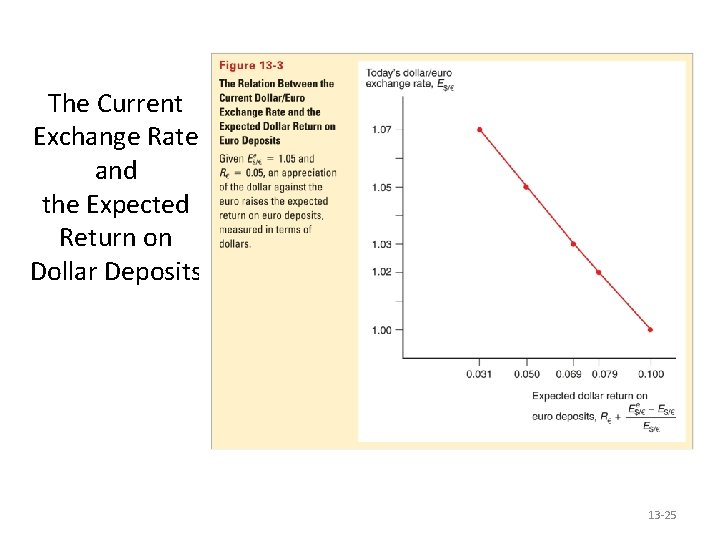The Current Exchange Rate and the Expected Return on Dollar Deposits 13 -25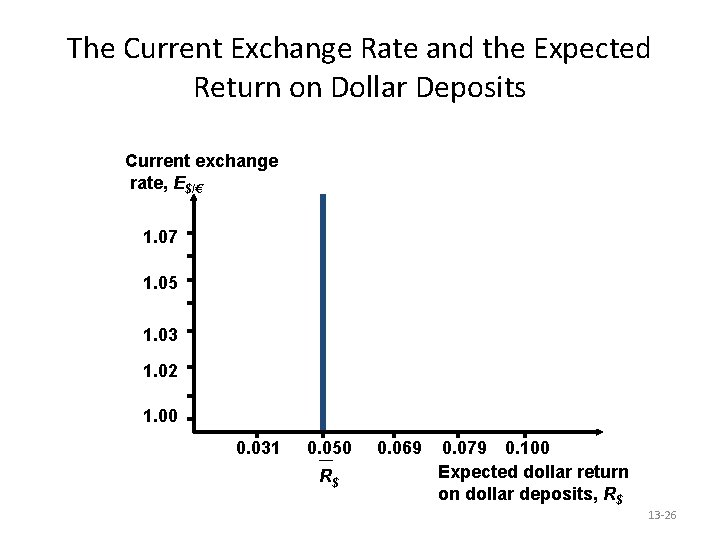The Current Exchange Rate and the Expected Return on Dollar Deposits Current exchange rate, E\$/€ 1. 07 1. 05 1. 03 1. 02 1. 00 0. 031 0. 050 R\$ 0. 069 0. 079 0. 100 Expected dollar return on dollar deposits, R\$ 13 -26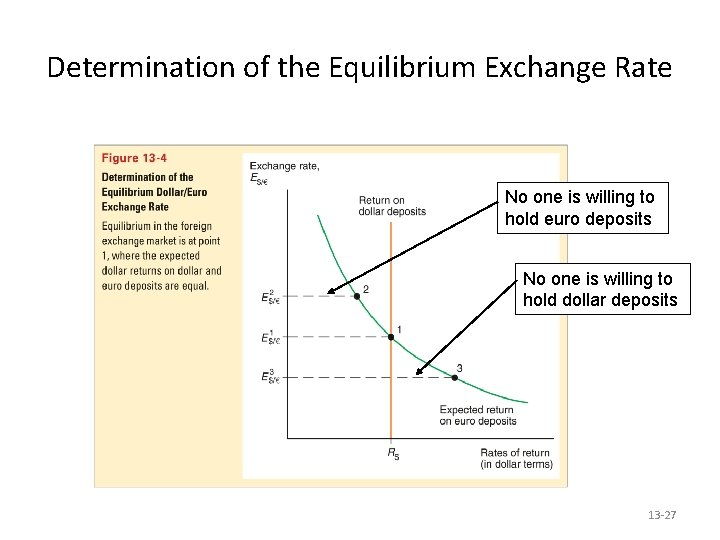Determination of the Equilibrium Exchange Rate No one is willing to hold euro deposits No one is willing to hold dollar deposits 13 -27The Market for Foreign Exchange • The effects of changing interest rates: – an increase in the interest rate paid on deposits denominated in a particular currency will increase the rate of return on those deposits. – This leads to an appreciation of the currency. – A rise in dollar interest rates causes the dollar to appreciate. – A rise in euro interest rates causes the dollar to depreciate. 13 -28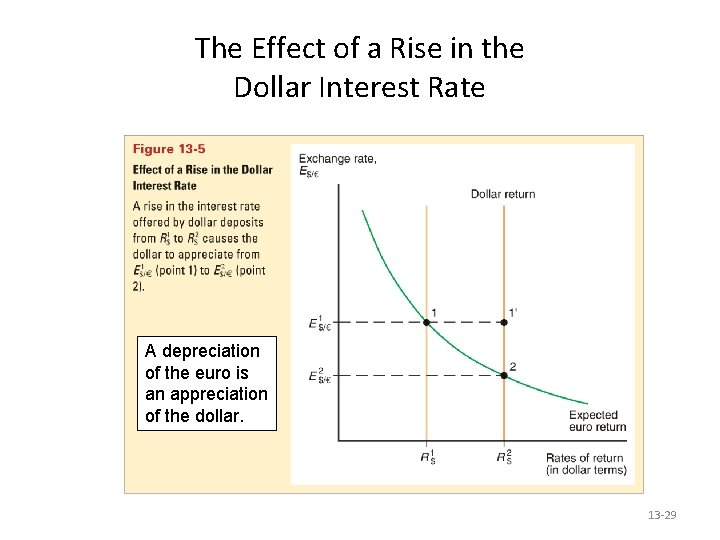The Effect of a Rise in the Dollar Interest Rate A depreciation of the euro is an appreciation of the dollar. 13 -29